Note: Please read the article carefully before proceeding! If in the process of using you encounter any errors, such as broken download links, slow loading blog, or unable to access a certain page on the blog ... then please inform me through the Contact page. Thanks!

Yes, it is very important to calculate the number of positive and negative numbers on Excel worksheet for you to work in accounting. In the previous tutorial, I showed you how to separate positive and negative numbers in Excel with the IF function in a very detailed and understandable way.

And to better assist you in perfecting your spreadsheets and reports with many different specific conditions today ....

.. I will continue to share with you another trick related to other positive and negative numbers, which is how to count and sum the values ​​of positive and negative numbers in Excel using COUNTIF and SUMIF The most specific.

### #first. How to count positive and negative numbers in Excel with the COUNTIF function

+ Step 1: Perform a negative number counting.

First you open the Excel file to handle up => here you click on the box to extract negative values.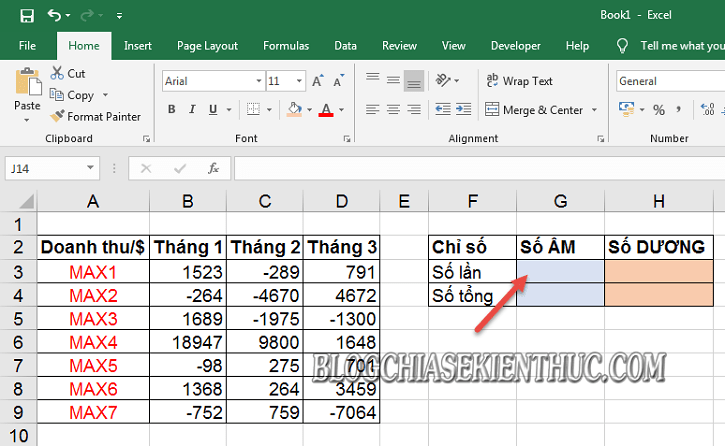+ Step 2: Now we will use the Countif function to count the number of repetitions of a value.

= COUNTIF( scan the selected area; condition) => then press F4 to fix formula => Enter to execute.

Apply it to the example to count smaller numbers `0` in the word region `B3:D9` we get:

= COUNTIF(\$ B \$ 3: \$ D \$ 9;"<0")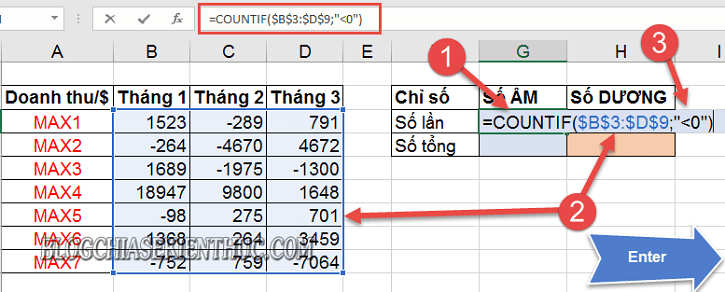And this is the result after we have applied the formula successfully.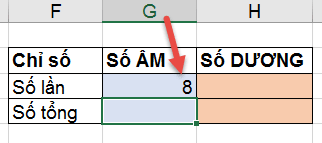+ Step 3: Counting positive numbers.

With the way to count positive numbers, you also enter the same formula, except in the condition, instead `“<0”`), then change the condition now `“>0”`) => okay `Enter`.

The formula to count positive numbers is: = COUNTIF( scan the selected area; condition> 0)

Applied to the example we have the following function:

= COUNTIF(\$ B \$ 3: \$ D \$ 9;"> 0")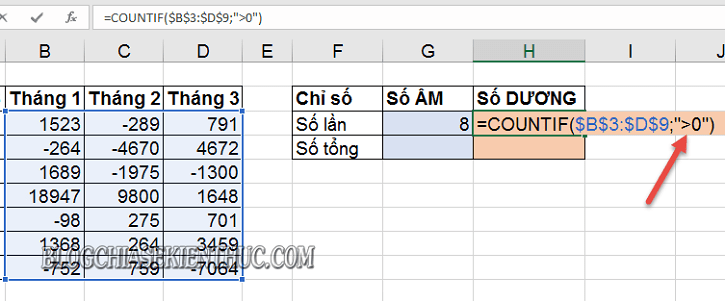To count the positive number results on the worksheet as shown below.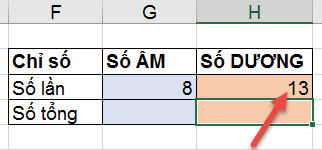### #2. How to calculate positive and negative totals with the SUMIF function

#### + Sum of negative numbers included in the spreadsheet

Similar to how to calculate the number of Yin and Yang numbers using the COUNTIF function. With the calculation, you also perform the same requirements with the SUM and IF functions (that is, the conditional sum function).

Formula to calculate conditional sum function: = SUMIF(Spreadsheet area, Condition)

Remember to use the F4 key to fix the worksheet area => then press Enter to perform.

Applied to the example we will get the function formula: = SUMIF(\$ B \$ 3: \$ D \$ 9; "<0”)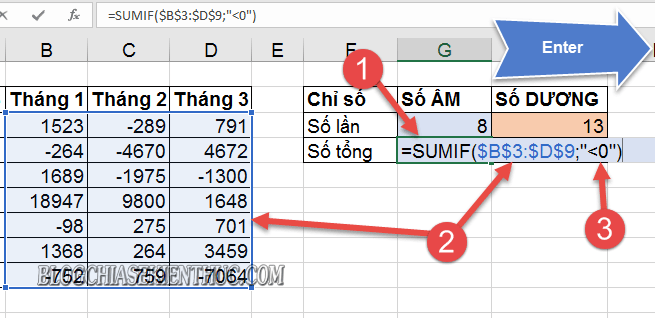After pressing Enter, we will get the total value of negative numbers on the worksheet as shown below.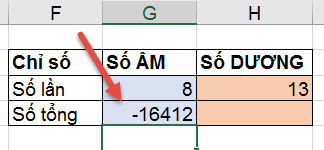#### + Sum of positive numbers included in the spreadsheet

Next to calculate the total value of positive numbers, then you use the same formula, just replace the word condition `“<0”` to `“>0”` is to be.

Specifically, we will get the following formula: = SUMIF(\$ B \$ 3: \$ D \$ 9; "> 0”)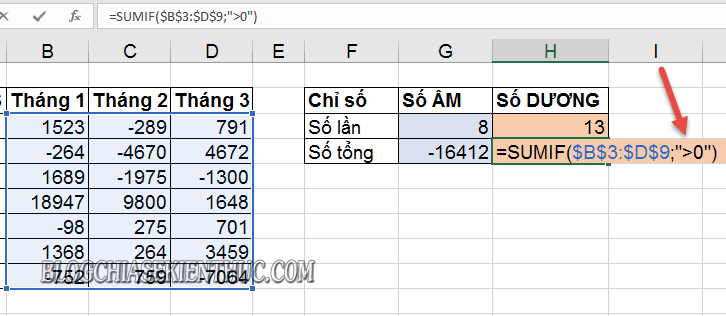The result is as shown below.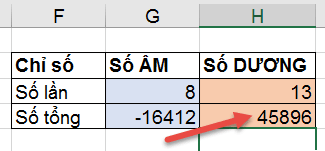### # 3. Epilogue

Okay, so I have just detailed instructions for you small tips on using the COUNTIT and SUMIF functions to Count and calculate the number of negative and positive numbers in Excel, given given conditions.

At this point, my article would like to pause. Hope this tip will be helpful to you. Good luck !

CTV: Luong Trung - Blogchiasekienthuc.com